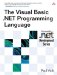# Object Data Type

 <  Day Day Up  >

The Object data type is a special type in the .NET Framework type system in that, unlike other types, a variable typed as Object can have values of any type assigned to it. For example, the following is valid code.

` Sub Main()   Dim o1, o2, o3 As Object   o1 = 5   o2 = "abc"   o3 = #8/23/70 4:30:23 AM# End Sub `

Why Object is such a special type is discussed in more detail in Chapter 13, Inheritance, but for now it is important to know only that an Object variable can hold any type, even reference types (which are discussed more in Chapter 9).

The default value of an Object variable is the literal Nothing , but Nothing is treated specially in the case of Object . When an Object variable containing Nothing is converted to another type, Nothing is converted to the default value of the target type. For example, the following code prints the number 0.

` Sub Main()   Dim o As Object   Dim i As Integer   i = 5   i = o   Console.WriteLine(i) End Sub `

The first assignment statement in the example assigns the value 5 to the variable i . The second statement assigns the value of the variable o to the variable i . Because o is initialized to Nothing , Nothing is converted to the default value of Integer , which is 0.

The Object type can be used in one of two ways. One way is to use the fact that Object can hold any kind of value to create very general code. For example, if you wanted to create a subroutine that accepted any kind of value and printed the type of that value, you could write the following:

` Sub PrintType(ByVal o As Object)   Console.WriteLine(o.GetType().ToString()) End Sub `

Or you could write a general program to add 1 to any kind of numeric type.

` Function AddOne(ByVal o As Object) As Object   If TypeOf o Is Integer Then     Return CInt(o) + 1   ElseIf TypeOf o Is Double Then     Return CDbl(o) + 1   ' Handle the other types   ...   Else     Throw New ArgumentException("Not a recognized value.")   End If End Function `

A second way of using Object is to use it avoid worrying about what type the variables in a program are by typing everything as Object . When writing a Visual Basic .NET program in this way, the programmer is taking advantage of loose typing . Although all variables in a Visual Basic .NET program have a type, by typing all variables as Object , the programmer is leaving it entirely up to the compiler and the Framework to figure out what type things are at runtime and ensure correct behavior. While this can be a simpler way to write programs, it has drawbacks. Because the types of variables are not known to the compiler, more work has to be done at runtime to figure out what the correct behavior is. For example, in the following program, since the variables a and b are typed as Integer , the compiler knows that it is doing Integer addition. The variables c and d , however, are typed as Object , so the compiler does not necessarily know what type of addition is being done until runtime.

` Dim a, b As Integer Dim c, d As Object a = 10 b = 20 a = a + b c = 10 d = 20 c = c + d `

## Style

In general, programmers are encouraged to use strong typing ”that is, to specify the exact type of all the variables declared in the program. Strong typing ensures that many logic errors are caught at compile time; it also produces faster and more efficient code. The Option Strict statement ensures that you use strong typing in your code.

 <  Day Day Up  >The Visual Basic .NET Programming Language
ISBN: 0321169514
EAN: 2147483647
Year: 2004
Pages: 173
Authors: Paul Vick

Similar book on Amazon